﻿ Designing Exercises to Determine the Structural Formula of Organic Compounds Based on the Experimental DataPublications are Open
Access in this journal
Article Versions
Export Article
• Normal Style
• MLA Style
• APA Style
• Chicago Style
Research Article
Open Access Peer-reviewed

### Designing Exercises to Determine the Structural Formula of Organic Compounds Based on the Experimental Data

C.C. Giac , P.H. Thanh, N.X. Ninh, N.T.D. Hang
World Journal of Chemical Education. 2017, 5(2), 23-28. DOI: 10.12691/wjce-5-2-1
Published online: April 07, 2017

### Abstract

This paper introduces the way to design exercises to determine the structural formula of organic compounds based on the experimental data. They are structure, physical properties, typical chemical properties, synthesis methods and application. From that the authors have built the scheme to design exercises. On that basis, the authors compiled some exercises which are used for teaching and learning organic chemistry in university and high school.

### 1. Introduction

Designing exercises is essential in teaching chemistry, an indispensable activity of chemistry teacher at the universities and high schools. Organic chemistry exercises have more interesting contents, special exercises for identified structural formula of organic compounds will require students to have a basic knowledge of organic chemistry such as stereochemistry, reaction mechanisms or organic synthesis, etc 1.

Exploiting the experimental data to determine the structure of organic compounds are important skills to help students practice on scientific research in the university. Solving this exercises will help students to form experimental thinking in teaching of chemistry and research of organic chemistry.

### 2. Method – Desining Exercises

In order to determine the structural formula of organic compounds must first identify the components of qualitative and quantitative elements present in organic compounds, mean that molecular formula. Since it is based on experimental data on the molecular structure, chemical and physical properties of organic compounds and synthetic methods, we can argue to determine correctly structural formula of the organic compounds 2.

General scheme to design exercises as shown below.

Summarizing some typical chemical properties in Table 1.

### 3. Sample Problems

Example 1

From pepper isolated alkaloid piperine A (C17H19NO3) is a neutral substance 3. Ozonolysis of compound A formed substances: ethanedial (ethane-1,2-dione), B, D. Hydrolysis of B formed OHC-COOH and six-member heterocyclic compounds piperidine (C5H11N). Given D reacts with concentrated solution of HI to form 4,5-dihydroxybenzandehyde. Identify structural formulae of A, B, D. How many stereoisomers of A are there?

Solution:

- Hydrolysis of B formed OHC-COOH and piperidine, inferred B to contain the O=C-N- bond (nitrogen atom belongs to six-member ring). Structural formula of B is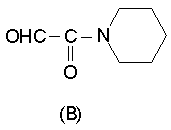- D reacts with HI to form 4,5-dihydroxybenzandehyde. Structural formula of D is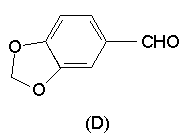- Ozonolysis of compound A formed ethanedial, inferred A contains the =CH-CH= group.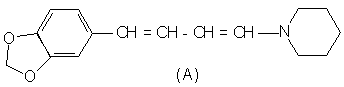- Compound A has 2 double bonds C=C, so there are 4 geometric isomers: ZZ, EE, ZE, EZ.

Example 2

Given an organic compound X is created by three chemical elements, has a molar mass of 282 g/mol. Mass analysis of X shows 17.02% oxygen. Complete combustion X formed moles of CO2 twice as much as moles of H2O. Let X reacts with excess (i-C3H7O)3Al / i-C3H7OH to form compound Y which has a molar mass of 288 g/mol. When reduction or oxidation ozonolysis to form the only compound Z which has a molar mass of 314 g/mol. Reduction Z by NaBH4, then the resultant product is oxidized with NaIO4 to form mixture of o-C6H4(CHO)2 and OHC(CH2)5CH(OH)(CH2)2CHO. On the other hand, treatment X with NaNH2 / DMF to form X1 (C18H18O3). Let X1 reacts with H2 / Pd, then heated resultant product with conc. H2SO4 to give X2 (C18H20O).

a) Draw the structural formula of X, Y, Z, X1 and X2.

b) Explain the formation mechanism of X1 from X.

Solution:

a) Burning X for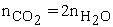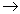The number of carbon atoms equal the number of hydrogen atoms.

Call the molecular formula of X is CxHxOz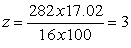MX = 13x + 16 x 3 = 282x = 18The molecular formula of X is C18H18O3.

For MY – MX = 288 – 282 = 6The molecular formula of Y compared to X to increase 6H after the Meerwein–Ponndorf–Verley (MPV) reduction 5, proving that X has 3 carbonyl groups (C = O).

We have transformed the following: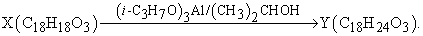The oxidation ozonolysis of X to form the only compound Z which has MZ – MX = 32Proving that oxidation ozonolysis of X which had gained more 2 oxygen atoms to form Z. Therefore, X has a double bond (C = C) in the ring and in this double bond is no longer hydrogen atom. The structural formula of X, Y, Z, X1 and X2 are determined by the following diagram:

b) Mechanism of forming X1 from X according to Michael addition reaction through 2 steps:

Step (1): NaNH2 attacks on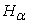to form carbanion.

Step (2): Carbanion attack on carbon to produce ring with 6 sides.

Example 3

Following is the 1H-NMR spectrum 6 for compound X of molecular formula C7H14O.

Propose a structural formula for compound X.

Solution:

From the 1H-NMR spectrum to the structural formula for compound X.

- Examine the molecular formula, calculate the degree of unsaturation (DU), and deduce what information you can about the presence or absence of rings or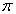- ponds. The molecular formula C7H14O that has DU = 1the compound contains 1 ring or 1- pond.

- Count the number of signals to arrive at a minimum number of different sets of hydrogens in the compound. There are three signals, therefore, three sets of equivalent hydrogens.

- Use the line of integration and the molecular formula to determine the number of hydrogens in each set. Reading from right to left, signals are in the ratio 9H : 3H : 2H.

- Examine the NMR spectrum for signals characteristic of the following types of hydrogens. Keep in mind that these are broad ranges and that hydrogens of each type may be shifted either farther upfield or farther downfield depending on details of molecular structure.

The singlet at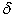1.01 is characteristic of a methyl group adjacent to an sp3 hybridized carbon. The singlets at2.11 and 2.32 are characteristic of alkyl groups adjacent to a carbonyl group.

- Examine splitting patterns for information about the number of nonequivalent neighboring hydrogens. All signals are singlets (s).

- Write a structural formula consistent with the previous information. The compound X is 4,4-dimethylpentan-2-one.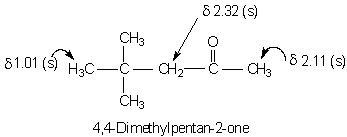Example 4

Safrole A, C10H10O2, a sweet-smelling liquid isolated from oil sassafrass, given the following properties:

- It does not dissolve in NaOH or give a color with FeCl3.

- It adds 1 eq of H2 on catalytic hydrogenation.

Reductive ozonolysis affords H2C=O and B (C9H8O3) that gives a positive Tollens’ test.

- Oxidation of A with KMnO4 gives an acid E (MW = 166) which gives no color with FeCl3.

- When E is refluxed with conc. HI, H2C=O and 3,4-dihydroxybenzoic acid are isolated and identified.

Deduce the structures of A, B and E.

Solution:

DU (degree of unsaturation) = 6, four of which are due to a benzene ring.

Since (A) does not dissolve in NaOH or give a color with FeCl3, it is not a phenol.

Formation of H2C=O on ozonolysis means that A has a chain with a terminal =CH2 grouping, and since B is an aldehyde (positive Tollens’ test), the grouping is –CH=CH2. The double bond accounts for the fifth DU.

E is a monocarboxylic acid formed by complete oxidation of the alkenyl side chain, and its molecular weight is 166.

No other C can be directly attached to the ring because it would have oxidized, giving a dicarboxylic acid.

The two remaining Os must be present as ether linkages probably present as a ring, the sixth DU, fused to the benzene ring. This is confirmed by isolating H2C=O and 3,4-dihydroxybenzoic acid on cleavage with HI. This fused ring is actually a stable acetal.

The molecular formula of E is C8H6O4. The acetal ring and the benzene ring account for seven Cs, leaving three Cs for the alkenyl side chain. The structures for A, B and E are: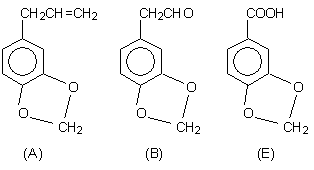Example 5

A pleasantly smelling, optically active compound F (MW = 186) 9. It does not react with Br2 in CCl4. Hydrolysis of F gives two optically active compounds, G, which is soluble in NaOH, and H. Compound H gives a positive iodoform test, and on warming with conc. H2SO4 gives I with no diastereomers. When the Ag+ salt of G is reacted with Br2, racemic J is formed. Optically active J is formed when H is treated with tosyl chloride (TsCl) and then NaBr. The IR spectrum of F shows a single C=O stretching peak. Give structures of F through J and explain your choices.

Solution:

F is a saturated monoester with MW = 186 (no Br2 reaction). We can logically determine the number of Cs and the molecular formula by subtracting the mass of the two Os and dividing the remainder by 14, the mass of CH2: (186 - 32)/14 = 11. To complete the mass there must be 22 Hs. The molecular formula is C11H22O2. The acid G has one more C than the alcohol H because it is degraded by one C in the Hunsdiecker reaction 7, 8 (RCOO-Ag+ + Br2) to J which is also made from H with no change in C content. H is a methyl carbinol, CH3CH(OH)R, because it gives a positive iodoform reaction and, in order to be chiral, must have at least four Cs. However, H has five Cs because the alkene, I, obtained on dehydration (warm conc. H2SO4), must have two Mes on one of the doubly bonded Cs to avoid cis-trans isomerism. I is CH3CH=C(CH3)2 with five Cs and G had six Cs. H is CH3CHOHCH(CH3)2 and is converted to J, CH3CHBrCH(CH3)2, through the tosylate, CH3CH(OTs)CH(CH3)2, with no change in configuration, by an SN2 reaction with Br-. Consequently, H and J have inverted configurations. The skeleton of the alkyl group of G is the same as H. Replacing Br of J by COOH gives the structure of G, (CH3)2CHCH(CH3)COOH. F is one of the four possible enantiomers of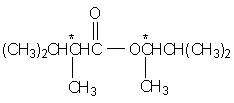### 4. Conclusions

Above are some examples of building exercises to determine the structure of organic compounds based on empirical data. Because empirical data on the structure is very rich so there are many forms of this type of exercise. In resolving the types of exercises will improve the students' thinking, especially the analysis of the reaction mechanism and the structure of organic compounds.

### Acknowledgements

We thank the anonymous reviewers of this paper for many very helpful suggestions.

### References

  Hass, M.A. Student-Directed learning in organic chemistry laboratory. J. Chem. Educ. 2000, 77(8), 1035-1038. In article View Article  Ram, P. Problem-based learning in undergraduate education. J. Chem. Educ. 1999, 76(8), 1122-1126. In article View Article  Giac C.C. The Diamonds in Chemistry. The Pedagogical University Publishing House, HaNoi, Vietnam, 2015, 1077-1129 In article  Raymond E. Davis, Regina Fray, Mickey Sarquis, Jerry L. Sarquis. Modern Chemistry. Holt, Rinehart and Winston, United States of America, 2009, 710-745. In article  Wolfgang Ponndorf (1926). “Der reversible Austausch der Oxydationsstufen zwischen Aldehyden oder Ketonen einerseits und primären oder sekundären Alkoholen anderseits”. Angewandte Chemie. 39 (5): 138-143. In article View Article  William H. Brown. Introduction to Organic Chemistry. Saunders College Publishing, 1997, 587-589. In article  Z. Wang, L. Zhu, F. Yin, Z. Su, Z. Li, C. Li (2012). Silver-Catalyzed Decarboxylative Chlorination of Aliphatic Carboxylic Acids, J. Am. Chem. Soc., 2012, 134, 4258-4263. In article View Article  Johnson, R. G.; Ingham, R. K. (1956). “The Degradation of Carboxylic Acid Salts by Means of Halogen - the Hunsdiecker Reaction”. Chem. Rev. 56 (2): 219-269. In article View Article  Estelle Meislich, Herbert Meislich, Jacob Sharefkin. 500 Organic Chemistry Questions. McGraw-Hill, America, 2013, 79-80. In articleThis work is licensed under a Creative Commons Attribution 4.0 International License. To view a copy of this license, visit http://creativecommons.org/licenses/by/4.0/